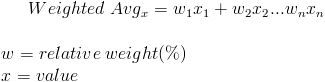# Weighted AverageThe weighted average formula is used to calculate the average value of a particular set of numbers with different levels of relevance. The relevance of each number is called its weight. The weights should be represented as a percentage of the total relevancy. Therefore, all weights should be equal to 100%, or 1.

The most common formula used to determine an average is the arithmetic mean formula. This formula adds all of the numbers and divides by the amount of numbers. An example would be the average of 1,2, and 3 would be the sum of 1 + 2 + 3 divided by 3, which would return 2. However, the weighted average formula looks at how relevant each number is. Say that 1 only happens 10% of the time while 2 and 3 each happen 45% of the time. The percentages in this example would be the weights. The weighted average would be 2.35.

The weighted average formula is a general mathematical formula, but the following information will focus on how it applies to finance.

## Use of Weighted Average Formula

The concept of weighted average is used in various financial formulas. Weighted average cost of capital (WACC) and weighted average beta are two examples that use this formula.

Another example of using the weighted average formula is when a company has a wide fluctuation in sales, perhaps due to producing a seasonal product. If the company would like to calculate the average of one of their variable expenses, the company could use the weighted average formula with sales as the weight to gain a better understanding of their expenses compared to how much they produce or sell.

## Example of Weighted Average Formula

A basic example of the weighted average formula would be an investor who would like to determine his rate of return on three investments. Assume the investments are proportioned accordingly: 25% in investment A, 25% in investment B, and 50% in investment C. The rate of return is 5% for investment A, 6% for investment B, and 2% for investment C. Putting these variables into the formula would bewhich would return a total weighted average of 3.75% on the total amount invested. If the investor had made the mistake of using the arithmetic mean, the incorrect return on investment calculated would have been 4.33%. This considerable difference between the calculations shows how important it is to use the appropriate formula to have an accurate analysis on how profitable a company is or how well an investment is doing.

New to Finance?